# Real numbers + quadratic equation - examples

1. PoolIf water flows into the pool by two inlets, fill the whole for 18 hours. First inlet filled pool 6 hour longer than second. How long pool is filled with two inlets separately?
2. Rectangle SSPerimeter of a rectangle is 268 cm and its diagonal is 99.3 cm. Determine the dimensions of the rectangle.
3. Euclid1Right triangle has hypotenuse c = 27 cm. How large sections cuts height hc=3 cm on the hypotenuse c?
4. Pumps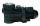The tank is filled with two pumps in 16 minutes. The first pump is filled in 30 minutes earlier than two one. How many minutes is filled with the first pump?
5. Hypotenuse and heightIn a right triangle is length of the hypotenuse c = 56 cm and height hc = 4 cm. Determine the length of both trangle legs.
6. CircleFrom the equation of a circle: ? Calculate the coordinates of the center of the circle S[x0, y0] and radius of the circle r.
7. Biquadratic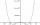By introducing a new variable solve biquadratic equation: ?
8. Resistors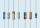Two resistors connected in series give the resulting resistance 216Ω and 48Ω in parallel. Determine the resistance of this resistors.
9. Factors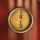Can the expression ? be factored into rational factors?
10. Equation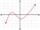Eequation f(x) = 0 has roots x1 = 64, x2 = 100, x3 = 25, x4 = 49. How many roots have equation f(x2) = 0 ?
11. Quadratic equationDetermine the numbers b, c that the numbers x1 = -1 and x2 = 3 were roots of quadratic equation: ?
12. Domains of functions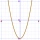F(x)=x2-7x and g(x)=5-x2 Domain of (fg)(x) is. .. . . Domain of (f/g)(x). ..

We apologize, but in this category are not a lot of examples.
Do you have an interesting mathematical example that you can't solve it? Enter it, and we can try to solve it.

To this e-mail address, we will reply solution; solved examples are also published here. Please enter e-mail correctly and check whether you don't have a full mailbox.

Looking for help with calculating roots of a quadratic equation?